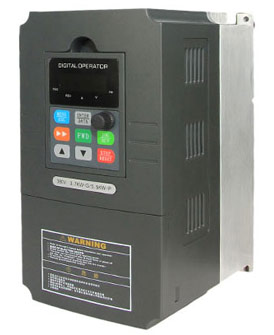Categories

# How VFDs save energy

Variable frequency drive (VFD) will capable of varying the output speed of a motor without the need for mechanical pulleys, thus reducing the number of mechanical components and overall maintenance. But the biggest advantage that a VFD has is the ability to save the user money through its inherit nature to save energy by consuming only the power that's needed.

A VFD will convert its incoming power, fixed voltage and frequency to variable voltage and frequency hence Vin x Iin x PFin = Vout x Iout x PFout. For a VFD operating at half speed and under full load, the input current is less than half of the output voltage. The secret is PF being a function of V and A. VFD helps in the level of harmonics content(displacement and distortion) which multiply each other to decrease efficiency; True PF = distortion PF x displacement FP. Hence VFD leverages on EFFICIENCY.Another important factor for Centrifugal application is the Law of AFFINITY: - Flow is proportional to shaft Speed. (Advance, high volumetric flow rate in less runtime),

1. Head (pressure) is proportional to square of shaft speed
2. Power is proportional to cube of shaft speed.

Hence PF, Efficiency, harmonics stability and Shaft speed find leverage in VFD that is best tied to a PLC for optimization. But don't forget the cost benefit factor of VFD in terms of energy mgt.

When induction motors run at less than 70% load its efficiency and power factor reduce drastically and energy is wasted. If the motors driving blowers and pumps are driven by VFDs and the speed is adjusted according to loads rather than dampers and throttle valves are used the efficiency and power factor improve considerably and hence energy is saved. Prima facie, the VFDs make use of one of the pump affinity laws, which states that power is directly proportional to the cube of speed. Therefore, from this law, you can deduce how a VFD saves energy by operating a motor and hence a pump at the desired flow rate, thereby reducing energy consumption.

Buy a VFD from GoHz Inc, 1hp VFD, 2hp VFD, 10hp VFD...